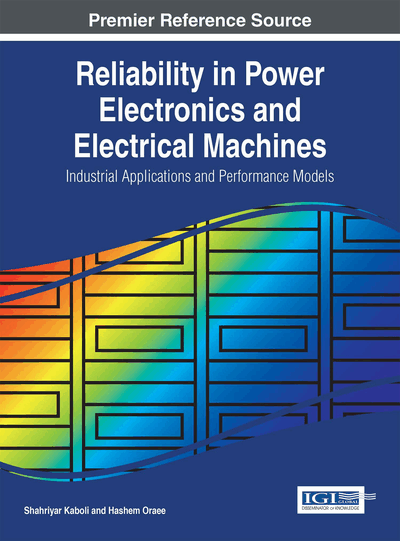# Thermal Analysis

DOI: 10.4018/978-1-4666-9429-3.ch004
Available
\$37.50
No Current Special Offers

## Abstract

Over temperature is one of the main reasons of failure in electric power converters. In addition, some of other failure factors such as dielectric breakdown act as over temperature in damaging process of a converter. In the previous chapter, it is emphasized that temperature factor is a key index in reliability calculation. Unlike fully electrical variables, thermal calculations require details of geometry of the system and its environment. In this chapter, thermal analysis as the most important factor in failure of converters is presented. Two main approaches for this goal are presented: numerical and lumped mode. Principles of these methods are described with various examples and a comparison is presented. Basic principles of thermal modeling are described and concept of sample node is explained. Methods for thermal management of an electric power converter are described. These methods are at both component and system levels and contain various heat transfer mechanisms like conduction and convection. Theoretical methods and practical considerations for heat sink selection and proper mounting are presented. Thermal insulation classes and various standards related to thermal management are expressed. Industrial samples are presented to show application of theoretical topics in real world.
Chapter Preview
Top

## Introduction: Failures Due To Thermal Problems

This chapter is considered as a support for reliability calculation as it is shown in Figure 1. Over temperature is one of the main reasons of failure in the systems. In the opposite of other failure factors (for example: dielectric breakdown), over temperature calculation is not performed easily as the analysis of other failure factors are taken place. Thus, in this chapter, temperature calculations of electrical machines and power electronic converters are explained.

Top

## Method Of Heat Transfer

There are three modes of heat transfer from a source with higher temperature to a region of lower temperature.

### Conduction

Conduction heat transfer occurs when there is a temperature gradient in a system. Energy is transferred from the high temperature region to the low temperature region. The energy transferring requires a material medium to occur. The heat transfer rate is proportional to the temperature gradient in the direction of the heat flow, the area and a property of the system.

### Convection

Another means of heat transfer is convection. In convection heat transfer, simply the heat is transferred away from the hot or cold surface. There are a number of concerns to arrive at a adequate analytical treatment of the problem. For example, for the case of a hot plate in front of fan, the velocity at which the air blows over the hot plate obviously influences the rate of heat transfer.

The third means of heat transfer is radiation. In contrast to the mechanism of conduction and convection, where energy transfer through a material medium is involved, heat may also be transferred through region where a perfect vacuum exists.

Top

## Thermal Analysis Using Finite Element Method

In mathematics, the finite element method (FEM) is a numerical technique for finding approximate solutions to boundary value problems for differential equations (Gorginpour, Jandaghi, & Oraee, 2011). It uses the calculation methods to minimize an error function and produce a stable solution. FEM encompasses all the methods for connecting many simple element equations over many small subdomains, named finite elements, to approximate a more complex equation over a larger domain.

### Structure of the Calculation

A typical work out of the method is dividing the domain of the problem into a collection of subdomains, with each subdomain represented by a set of element equations to the original problem, systematically recombining all sets of element equations into a global system of equations for the final calculation (Boglietti, Cavagnino, Staton, Shanel, Martin, & Mejuto, 2009). The global system of equations has known solution techniques, and can be calculated from the initial values of the original problem to obtain a numerical answer. The subdivision of a whole domain into simpler parts has several advantages:

• Accurate representation of complex geometry

• Inclusion of dissimilar material properties

• Easy representation of the total solution

• Capture of local effects.

Numerical methods provide a prediction of heat flows. What this means is that it will give a visual or post-processed result of a simulation but the quantitative or absolute accuracy of the result is sensitive to the inclusion and accuracy of the appropriate parameters 1. This method is more appropriate for precise modeling to optimization (Wei, Ghosh, Velusamy, Sankaranarayanan, Skadron, & Stan, 2006). Numerical methods can give an insight into patterns that are difficult, expensive or impossible to study using experimental methods. Experiments can give a quantitative description of flow phenomena using measurements for one quantity at a time, at a limited number of points and time instances. If a full scale model is not available or not practical, scale models or dummy models can be used. The experiments can have a limited range of problems and operating conditions. Simulations can give a prediction of flow phenomena using software for all desired quantities, with high resolution in space and time and virtually any problem and realistic operating conditions.

## Complete Chapter List

Search this Book:
Reset